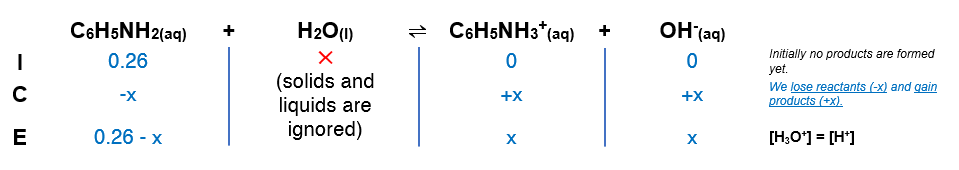# Problem: What is the pH of 0.26 M aniline?

###### FREE Expert Solution

Kb of aniline (C6H5NH2) is 4.3x10-10

(1) Setup an ICE chart(2) Setup Kb expression

$\overline{){{\mathbf{K}}}_{{\mathbf{b}}}{\mathbf{=}}\frac{\mathbf{products}}{\mathbf{reactants}}{\mathbf{=}}\frac{\mathbf{\left[}{\mathbf{OH}}^{\mathbf{-}}\mathbf{\right]}\mathbf{\left[}{\mathbf{C}}_{\mathbf{6}}{\mathbf{H}}_{\mathbf{5}}{{\mathbf{NH}}_{\mathbf{3}}}^{\mathbf{+}}\mathbf{\right]}}{\mathbf{\left[}{\mathbf{C}}_{\mathbf{6}}{\mathbf{H}}_{\mathbf{5}}{\mathbf{NH}}_{\mathbf{2}}\mathbf{\right]}}}$

Solving for x = [OH-]

88% (226 ratings)###### Problem Details

What is the pH of 0.26 M aniline?

Frequently Asked Questions

What scientific concept do you need to know in order to solve this problem?

Our tutors have indicated that to solve this problem you will need to apply the Weak Bases concept. If you need more Weak Bases practice, you can also practice Weak Bases practice problems.

What professor is this problem relevant for?

Based on our data, we think this problem is relevant for Professor Sanders' class at QC CUNY .

What textbook is this problem found in?

Our data indicates that this problem or a close variation was asked in Chemistry: The Molecular Nature of Matter and Change - Silberberg 8th Edition. You can also practice Chemistry: The Molecular Nature of Matter and Change - Silberberg 8th Edition practice problems.